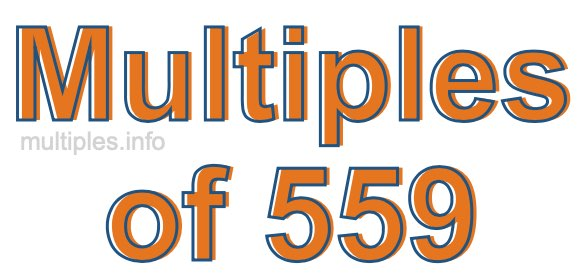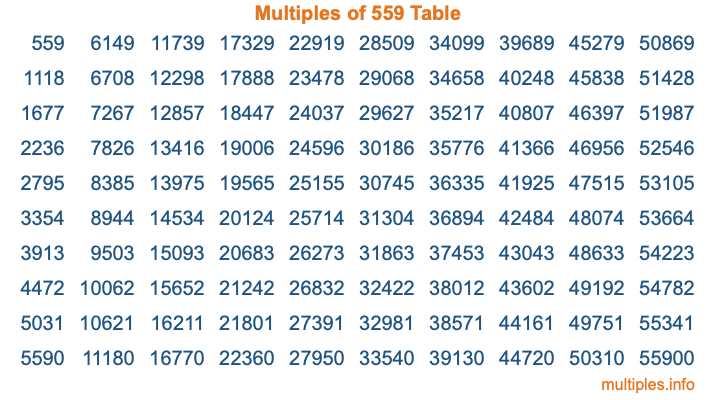Multiples of 559Welcome to the Multiples of 559 page. Here we will first teach you everything you will ever need to know about the multiples of 559, and then give you a study guide summary of everything we taught you to make sure you remember it all. Use this page to look up facts and learn information about the multiples of 559. This page will make you a multiples of five hundred fifty-nine expert!

Definition of Multiples of 559
Multiples of 559 are all the numbers that when divided by 559 equal an integer. Each of the multiples of 559 are called a multiple. A multiple of 559 is created by multiplying 559 by an integer.

Therefore, to create a list of multiples of 559, you start with 1 multiplied by 559, then 2 multiplied by 559, then 3 multiplied by 559, and so on for as long as you want. Thus, the list of the first five multiples of 559 is 559, 1118, 1677, 2236, and 2795. To see a larger list of multiples of 559, see the printable image of Multiples of 559 further down on this page. We also have a category where you can choose any nth multiple of 559.

Multiples of 559 Checker
The Multiples of 559 Checker below checks to see if any number of your choice is a multiple of 559. In other words, it checks to see if there is any number (integer) that when multiplied by 559 will equal your number. To do that, we divide your number by 559. If the the quotient is an integer, then your number is a multiple of 559.

Is  a multiple of 559?

Least Common Multiple of 559 and ...
A Least Common Multiple (LCM) is the lowest multiple that two or more numbers have in common. This is also called the smallest common multiple or lowest common multiple and is useful to know when you are adding our subtracting fractions. Enter one or more numbers below (559 is already entered) to find the LCM.

Check out our LCM Calculator if you need more details about the Least Common Multiple or if you need the LCM for different numbers for adding and subtraction fractions.

nth Multiple of 559
As we stated above, 559 is the first multiple of 559, 1118 is the second multiple of 559, 1677 is the third multiple of 559, and so on. Enter a number below to find the nth multiple of 559.

th multiple of 559

Multiples of 559 vs Factors of 559
559 is a multiple of 559 and a factor of 559, but that is where the similarities end. All postive multiples of 559 are 559 or greater than 559. All positive factors of 559 are 559 or less than 559.

Below is the beginning list of multiples of 559 and the factors of 559 so you can compare:

Multiples of 559: 559, 1118, 1677, 2236, 2795, etc.

Factors of 559: 1, 13, 43, 559

As you can see, the multiples of 559 are all the numbers that you can divide by 559 to get a whole number. The factors of 559, on the other hand, are all the whole numbers that you can multiply by another whole number to get 559.

It's also interesting to note that if a number (x) is a factor of 559, then 559 will also be a multiple of that number (x).

Multiples of 559 vs Divisors of 559
The divisors of 559 are all the integers that 559 can be divided by evenly. Below is a list of the divisors of 559.

Divisors of 559: 1, 13, 43, 559

The interesting thing to note here is that if you take any multiple of 559 and divide it by a divisor of 559, you will see that the quotient is an integer.

Multiples of 559 Table
Below is an image of the first 100 multiples of 559 in a table. The table is in chronological order, column by column. The first column has the first ten multiples of 559, the second column has the next ten multiples of 559, and so on.The Multiples of 559 Table is also referred to as the 559 Times Table or Times Table of 559. You are welcome to print out our table for your studies.

Negative Multiples of 559
Although not often discussed or needed in math, it is worth mentioning that you can make a list of negative multiples of 559 by multiplying 559 by -1, then by -2, then by -3, and so on, to get the following list of negative multiples of 559:

-559, -1118, -1677, -2236, -2795, etc.

Multiples of 559 Summary
Below is a summary of important Multiples of 559 facts that we have discussed on this page. To retain the knowledge on this page, we recommend that you read through the summary and explain to yourself or a study partner why they hold true.

There are an infinite number of multiples of 559.

A multiple of 559 divided by 559 will equal a whole number.

559 divided by a factor of 559 equals a divisor of 559.

The nth multiple of 559 is n times 559.

The largest factor of 559 is equal to the first positive multiple of 559.

559 is a multiple of every factor of 559.

559 is a multiple of 559.

A multiple of 559 divided by a divisor of 559 equals an integer.

559 divided by a divisor of 559 equals a factor of 559.

Any integer times 559 will equal a multiple of 559.

Multiples of a Number
Here you can get the multiples of another number, all with the same attention to detail as we did for multiples of 559 on this page.

Multiples of
Multiples of 560
Did you find our page about multiples of five hundred fifty-nine educational? Do you want more knowledge? Check out the multiples of the next number on our list!